## Using the Henyey-Greenstein distribution to model bulk scattering

Category: Product NewsThis blog post demonstrates how to use a provided user-defined DLL to model Henyey-Greenstein bulk scattering in Non-Sequential Mode. In the blog post, we will provide a brief overview of the theory behind the model and will show two examples of analysis of a system that is utilizing the DLL.

Introduction

The Henyey-Greenstein model describes the angular distribution of light scattered by small particles. This model has been applied to numerous situations, ranging from the scattering of light by biological tissue (1) to scattering by interstellar dust clouds.(2)

A user-defined DLL is included with the OpticStudio installation which allows users to apply this bulk scattering model to any non-sequential volume. In this post, the "Henyey-Greenstein-bulk.dll" will be used to show the angular and power distribution of the Henyey-Greenstein model.

Henyey-Greenstein bulk scatter model

The Henyey-Greenstein model describes the angular distribution of light scattered by small particles. In this model, the angular distribution of scattered light is given by:The parameter g characterizes the distribution. For g = 0, the model describes a material with a uniform probability of scattering at all angles, and as g approaches unity, the distribution becomes highly peaked around θ = 0 degrees.  The angle θ is defined as the angle of the scattered ray concerning the specular ray; θ = 0 degrees refers to scattering along the specular ray in the forward direction, and θ = 180 degrees to scattering along the specular ray in the backward direction.

The distribution of power in the Henyey-Greenstein model

We will now run throuhg an example illustrating the implementation of the Henyey-Greenstein model in OpticStudio. This system consists of a Source Ray launching rays at normal incidence to a Rectangular Volume in which the Henyey-Greenstein scattering distribution has been applied. This distribution is applied to the volume by specifying a user-defined DLL.

The settings in Object Properties...Volume Physics control what type of bulk scattering model to apply to the Rectangular Volume. In this example, the "Model" is DLL Defined Scattering, and the selected DLL file is “Henyey-Greenstein-bulk.DLL." This DLL and the corresponding source code are located in the folder "{Zemax}\DLL\BulkScatter."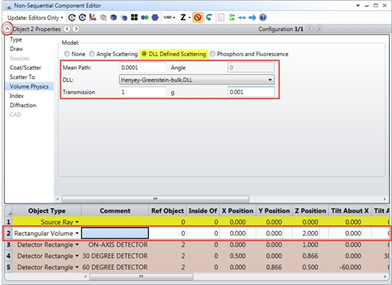Inputs to the DLL are the "Transmission" (which describes how much of the input power is attenuated during scattering) and the parameter "g" from the equation above. To measure scattered rays at various angles, in the example system three small detectors have been placed a uniform distance away from the volume, at angles of 0, 30, and 60 degrees concerning the incident ray angle:Note that the above screenshot shows an NSC 3D Layout without scattering turned on, so we’re only seeing the specular path. Before analyzing the results with scattering turned on, we can expand the Object Properties.Source for the Source Ray. You will notice that the system has been set up to explicitly provide only one instance of scattering per incident ray:The default Bulk Scatter setting is "Many," which means that the rays may scatter multiple times within the media. If “Once” is selected, each branch of a ray can only bulk scatter once. If a ray splits before scattering, for example at the interface of the scattering volume, then each of the child rays may scatter, since each child’s branch is scattering for the first time. If “Never” is selected, then no bulk scattering will occur for rays from this source.

Scattering can be observed in the NSC 3D Layout by checking Scatter NSC Rays in the Layout settings: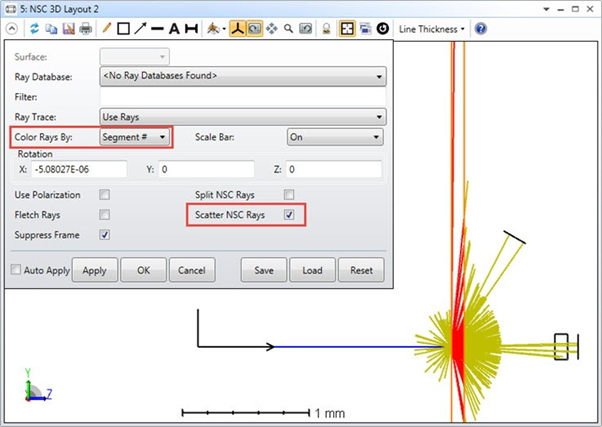Many of the rays miss the detectors, and these rays are only drawn a short distance to show their direction.  To change the distance these rays are drawn, see the “Missed Ray Draw Distance” under System Explorer...Non-Sequential: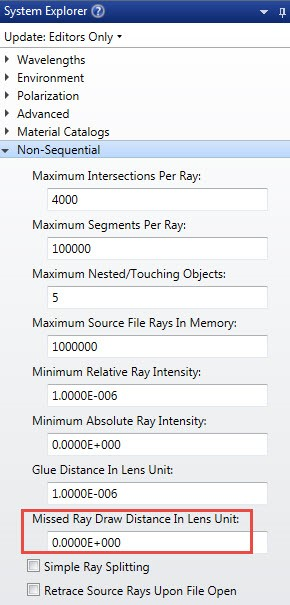This parameter is the distance to draw the ray segments that miss all objects. If zero, OpticStudio will select a default value for this parameter when drawing missed rays.

We can use Filter strings to analyze just the rays which scatter in the Rectangular Volume and hit the Detector rectangle. The Filter string defines a “test” that rays must pass before they are drawn or displayed. The Filter string syntax consists of logical operations between commands or flags that indicate if the ray has hit, missed, reflected, refracted, scattered, diffracted, or ghost reflected from an object. A full list of Filter string commands can be found in the OpticStudio Help Files: "The Setup Tab>Editors Group (Setup Tab)>Non-sequential Component Editor>Non-sequential Overview>The Filter String."

Here are the flags we will use:

• Bn: Show rays which bulk scattered inside of object #n.

• Hn: Show rays that hit object #n.

We can combine these Filter strings using the following operators:

• &: Logical AND. Both flags on either side of the & symbol must be TRUE for the AND operation to return TRUE.

• |: Logical OR. If either of the flags is TRUE OR returns TRUE.

Therefore, to show rays that were bulk scattered within the Rectangular Volume (Object #2) and hit one of the detectors (Objects #3, #4, #5) we can use the Filter string “B2 & (H3 | H4 | H5)." This Filter string may be applied to Layout rays in the NSC 3D Layout or the Shaded Model: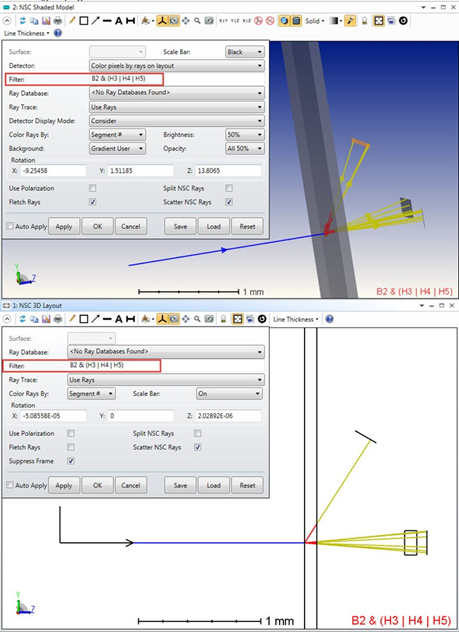Filter strings may be applied to Analysis Rays in the Ray Trace Control when the results are saved to Ray Database.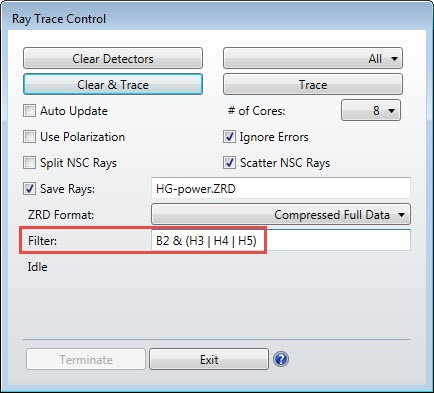The distribution of power landing on each detector is then measured for a case in which 5,000,000 Analysis Rays are launched with a total power of 1 W (and a transmission factor of unity). The results for "g" = 0.001 and "g" = 0.5 and shown in the table below:The Mean Path (mean free path) was set to 0.0001 mm, which is small relative to the 0.1 mm thickness of the volume. The measured OpticStudio values reproduce those results derived from the theoretical model within statistical error, as we would expect for a case in which each ray is only allowed to scatter once (the results will vary from ray trace to ray trace due to statistics, so you will get different – but very similar – numbers).

The measured values in the table above can be read from the individual detector viewers:Note: If "g" = 0 is specified as an input, the actual value of "g" used in the calculations is 10-4. This is due to a singularity in the calculations which arises when "g" = 0.  Results obtained for very small values of "g" are nearly identical to those expected for "g" = 0, indicating that this approximation is sufficiently accurate.

Author: